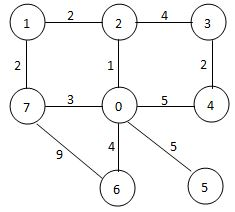## 在线测试传送门：PAT（甲级）2021年春仿真卷

7-4 Recycling of Shared Bicycles (30 分)
There are many spots for parking the shared bicycles in Hangzhou. When some of the bicycles are broken, the management center will receive a message for sending a truck to collect them. Now given the map of city, you are supposed to program the collecting route for the truck. The strategy is a simple greedy method: the truck will always move to the nearest spot to collect the broken bicycles. If there are more than one nearest spot, take the one with the smallest index.

Input Specification:
Each input file contains one test case. For each case, the first line contains two positive integers: N (≤ 200), the number of spots (hence the spots are numbered from 1 to N, and the management center is always numbered 0), and M, the number of streets connecting those spots. Then M lines follow, describing the streets in the format:

S1 S2 Dist

where S1 and S2 are the spots at the two ends of a street, and Dist is the distance between them, which is a positive integer no more than 1000. It is guaranteed that each street is given once and S1 is never the same as S2.

Output Specification:
For each case, first print in a line the sequence of spots in the visiting order, starting from 0. If it is impossible to collect all the broken bicycles, output in the second line those spots that cannot be visited, in ascending order of their indices. Or if the job can be done perfectly, print in the second line the total moving distance of the truck.

All the numbers in a line must be separated by 1 space, and there must be no extra space at the beginning or the end of the line.

Sample Input 1:

7 10
0 2 1
0 4 5
0 7 3
0 6 4
0 5 5
1 2 2
1 7 2
2 3 4
3 4 2
6 7 9Sample Output 1:

0 2 1 7 6 3 4 5
33


Sample Input 2:

7 8
0 2 1
0 4 5
0 7 3
1 2 2
1 7 2
2 3 4
3 4 2
6 5 1


Sample Output 2:

0 2 1 7 3 4
5 6


## AC代码

#include <bits/stdc++.h>
using namespace std;
const int N=210,inf=0x3f3f3f3f;
int n,m,dp[N][N];
bool vis[N];
void floyd() // 求两点间最短路
{
// 无负权边，可初始化自己到自己距离为0
for(int i=0;i<=n;i++)
dp[i][i]=0;
for(int k=0;k<=n;k++) // 中间点k的遍历一定要放在第一层循环！
for(int i=0;i<=n;i++)
for(int j=0;j<=n;j++)
if(i!=j)dp[i][j]=min(dp[i][j],dp[i][k]+dp[k][j]);
}
vector<int>ans;
int sum=0; // 记录最短路径和
void dfs(int u)
{
ans.push_back(u);
vis[u]=1;
int v=-1,mi=inf;
for(int i=1;i<=n;i++)
{
if(i!=u&&!vis[i]&&dp[u][i]<mi) // mi是当前找到的最短路，注意i!=u
{
mi=dp[u][i];
v=i;
}
}
if(v!=-1)
{
sum+=mi;
dfs(v);
}
}
int main()
{
ios::sync_with_stdio(false);
cin>>n>>m;
int x,y,z;
memset(dp,inf,sizeof(dp));
for(int i=1;i<=m;i++)
{
cin>>x>>y>>z;
dp[x][y]=dp[y][x]=z;
}
floyd();
dfs(0);
int sz1=ans.size();
for(int i=0;i<sz1;i++)
i==sz1-1?printf("%d\n",ans[i]):printf("%d ",ans[i]);
vector<int>t;
for(int i=1;i<=n;i++)
if(!vis[i])t.push_back(i);
int sz2=t.size();
if(sz2)
{
for(int i=0;i<sz2;i++)
i==sz2-1?printf("%d\n",t[i]):printf("%d ",t[i]);
}
else printf("%d\n",sum);
return 0;
}